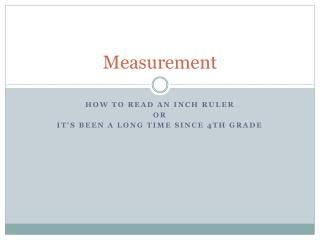DownloadDownload PresentationMeasurement

# Measurement

Download Presentation## Measurement

- - - - - - - - - - - - - - - - - - - - - - - - - - - E N D - - - - - - - - - - - - - - - - - - - - - - - - - - -
##### Presentation Transcript

1. Measurement HOW TO READ AN INCH RULER OR It’s been a long time since 4th grade

2. What measurement is this?

3. Answer: ½ inch

4. What you need to know This is 1/16 inch ruler.We know this because it has 16 white spaces in one inch.

5. What you need to know This is 1/16 inch ruler.We know this because it has 16 white spaces in one inch. This slide shows one inch.

6. Answer: 1/2 This is 1/16 inch ruler.We know this because it has 16 white spaces in one inch. This slide shows one inch. The size of the line it is pointing to gives you a hint as to what the measurement is. The longer the line the bigger the measurement. 1/2

7. Answer: 1/2 This is 1/16” ruler—we know this because it has 16 white spaces in it it shows one inch The size of the line it is pointing to gives you a hint as to what the measurement is The longest line on a ruler shows the inch mark, next longest shows the ½ inch mark, next longest shows the ¼. etc Fractions must always be reduced i.e. 1/8 not 2/16 1/8

8. What measurement is this

9. Answer: ¼ inch

10. What measurement is this

11. Answer: 1/8 inch

12. What measurement is this

13. Answer: ¾ inch

14. What measurement is this

15. Answer: 7/8 inch

16. What measurement is this

17. Answer: 1/2 inch

18. What measurement is this

19. Answer: 5/8 inch

20. What measurement is this

22. What measurement is this

23. Answer: 9/16 inch

24. Measurement HOW TO READ A pica gauge OR WOW! look at me acting like a graphic designer

25. Measuring with points and picas

26. Points converted to picas • Measurements: • 12 points =1 pica • 6 picas =1 inch • 72 points =1 inch • ½ pica= 6 points • A standard US letter size page is 8.5 by 11 inchesor 51 by 66 picas. p12 1p

27. Picas converted to inches • Measurements: • 12 points =1 pica • 6 picas =1 inch • 72 points =1 inch • ½ pica= 6 points • A standard US letter size page is 8.5 by 11 inchesor 51 by 66 picas. 6p 1 inch

28. Points converted to inches • Measurements: • 12 points =1 pica • 6 picas =1 inch • 72 points =1 inch • ½ pica= 6 points • A standard US letter size page is 8.5 by 11 inchesor 51 by 66 picas. 12 24 36 48 60 72 points 72 points or I inch

29. points | picas • Measurements: • 12 points =1 pica • 6 picas =1 inch • 72 points =1 inch • ½ pica= 6 points • A standard US letter size page is 8.5 by 11 inchesor 51 by 66 picas. 6 12 18 24 points ½ pica or 6 points

30. What measurement is this

31. Answer 8p9 | 8 picas 9 points

32. What measurement is this

33. Answer 1p | 1 picas 0 points

34. What measurement is this

35. Answer 1p6 | 1 picas 6 points

36. What measurement is this

37. Answer 2p3 | 2 picas 3 points